International
Tables for
Crystallography
Volume B
Reciprocal space
Edited by U. Shmueli

International Tables for Crystallography (2006). Vol. B. ch. 2.1, p. 203   | 1 | 2 |

## Section 2.1.7.4. Fourier versus Hermite approximations

U. Shmuelia* and A. J. C. Wilsonb

aSchool of Chemistry, Tel Aviv University, Tel Aviv 69 978, Israel, and bSt John's College, Cambridge, England
Correspondence e-mail:  ushmueli@post.tau.ac.il

#### 2.1.7.4. Fourier versus Hermite approximations

| top | pdf |

As noted in Section 2.1.8.7below, the Fourier representation of the probability distribution ofis usually much better than the particular orthogonal-function representation discussed in Section 2.1.7.3. Many, perhaps most, non-ideal centric distributions look like slight distortions of the ideal (Gaussian) distribution and have no resemblance to a cosine function. The empirical observation thus seems paradoxical. The probable explanation has been pointed out by Wilson (1986b). A truncated Fourier series is a best approximation, in the least-squares sense, to the function represented. The particular orthogonal-function approach used in equation (2.1.7.5), on the other hand, is not a least-squares approximation to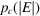, but is a least-squares approximation to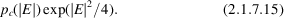The usual expansions (often known as Gram–Charlier or Edgeworth) thus give great weight to fitting the distribution of the (compararively few) strong reflections, at the expense of a poor fit for the (much more numerous) weak-to-medium ones. Presumably, a similar situation exists for the representation of acentric distributions, but this has not been investigated in detail. Since the centric distributionsoften look nearly Gaussian, one is led to ask if there is an expansion in orthogonal functions that (i) has the leading termand (ii) is a least-squares (as well as an orthogonal-function)2 fit to. One does exist, based on the orthogonal functions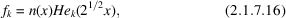where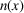is the Gaussian distribution (Myller-Lebedeff, 1907). Unfortunately, no reasonably simple relationship between the coefficients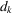and readily evaluated properties ofhas been found, and the Myller-Lebedeff expansion has not, as yet, been applied in crystallography. Although Stuart & Ord (1994, p. 112) dismiss it in a three-line footnote, it does have important applications in astronomy (van der Marel & Franx, 1993; Gerhard, 1993).

### ReferencesGerhard, O. E. (1993). Line-of-sight velocity profiles in spherical galaxies: breaking the degeneracy between anisotropy and mass. Mon. Not. R. Astron. Soc. 265, 213–230.Google ScholarMarel, R. P. van der & Franx, M. (1993). A new method for the identification of non-Gaussian line profiles in elliptical galaxies. Astrophys. J. 407, 525–539.Google ScholarMyller-Lebedeff, W. (1907). Die Theorie der Integralgleichungen in Anwendung auf einige Reihenentwicklungen. Math. Ann. 64, 388–416.Google ScholarStuart, A. & Ord, K. (1994). Kendall's advanced theory of statistics. Vol. 1. Distribution theory, 6th ed. London: Edward Arnold.Google ScholarWilson, A. J. C. (1986b). Fourier versus Hermite representations of probability distributions. Acta Cryst. A42, 81–83.Google Scholar